Courses

# Previous Year Questions - Pair of Linear Equations in Two Variables Class 10 Notes | EduRev

## Class 10 : Previous Year Questions - Pair of Linear Equations in Two Variables Class 10 Notes | EduRev

The document Previous Year Questions - Pair of Linear Equations in Two Variables Class 10 Notes | EduRev is a part of the Class 10 Course Mathematics (Maths) Class 10.
All you need of Class 10 at this link: Class 10

Short Answer Type Questions

Q.1. Find c if the system of equations    [CBSE 2019 (30/1/1)]
cx + 3y + (3 - c) — 0; 12x + cy - c = 0 has infinitely many solutions.

Ans: Given system of equations,
cx + 3y + (3 - c) = 0 and 12x + cy - c = 0
For infinite solutions, we know that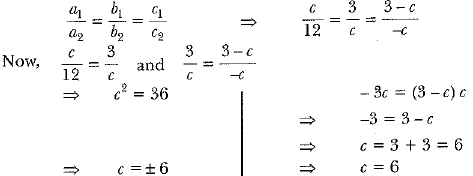∴ c = 6, for infinitely many solutions.

Q.2. Solve the following pair of linear equations.    [CBSE 2019 (30/3/3)3]
3x + 4y = 10 and 2x - 2y = 2

Ans: Given equations
3x + 4y = 10 ...(i)
2x - 2y = 2  ...(ii)
Multiply (i) by 2 and (ii) by 3, we get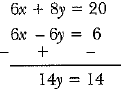⇒ y = 1   ∴ 2x - 2(1) = 2  ⇒2x = 4 ⇒ x = 2

Q.3. The larger of two supplementary angles exceeds the smaller by 18°. Find the angles.    [CBSE 2019 (30/2/1)]
Ans: Let the larger supplementary angle be x.
∴ Other angle = 180 - x
According to the question,
x = 180 - x + 18°
2x = 198°
x = 99°
∴ Supplementary angles are 99°, 81°.

Q.4. A father's age is three times the sum of the ages of his two children. After 5 years his age will be two times the sum of their ages. Find the present age of the father.    [ CBSE. 2019 (30/1/1)]
Ans: Let father's age be x years and the sum of ages of his children's ages be y years.
∴ x = 3y ...(i)
After 5 years Father’s age = (x + 5) years
Sum of his children’s age = y + 5 + 5 =( y + 10) years
∴ According to question,
x + 5 = 2(y + 10)
x + 5 = 2) + 20
⇒ 3y + 5 = 2y + 20  ⇒3y - 2) = 20 - 5
⇒ y = 15 [From (i)]
Putting) = 15 in equation (i), we get
x = 3y = 3 x 15 = 45
∴ Father’s age = 45 years

Q.5. A fraction becomes 1/3  when 2 is subtracted from the numerator and it becomes 1/2 when 1 is 3 subtracted from its denominator. Find the fraction.

[CBSE 2019 (30/1/1]
Ans:
Let numerator of fraction be x and denominator be y.
∴ Fraction = x/y
According to the question,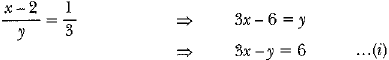Again,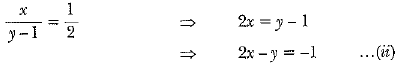From equation (i) and (ii), we have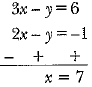⇒ Now, putting x = 7 in equation (i), we have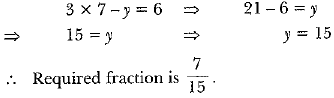Q.6. Find the value(s) of k so that the pair of equations x + 2y = 5 and 3x + ky +15 = 0 has a unique solution.    [CBSE, Allahabad 2019]
Ans: x + 2y - 5 = 0 ...(i)
3x + ky +15 = 0 ...(ii)
a1 = 1, b1 = 2, c1 = - 5
a2 = 3, b2 = k, c2 = 15
For unique solution,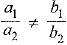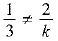⇒ k ≠ 6
So, the given system of equations is consistent with a unique solution for all values of k other than 6.

Q.7. In Fig., ABCD is a rectangle. Find the values of x and y.    [CBSE 2018]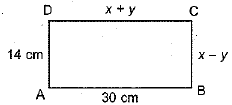Ans: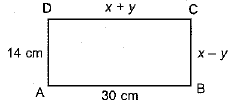We know that opposite sides of a rectangular are equal. So,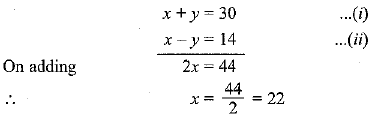Putting x = 22 in eq. (i), we have
22 + y = 30
⇒ y = 30 - 22 - 8
∴ x = 22 cm and y = 8 cm

Long Answer Type Questions

Q.1. For what values of m and n the following system of linear equations has infinitely many solutions.
3x + 4y = 12
(m + n)x + 2 (m - n)y = 5m - 1    [CBSE 2018 (C)]
Ans: 3x + 4y = 12 = 0 ...(i)
(m + n)x + 2(m - n)y = 5 m - 1 ...(ii)
System of linear equations has infinitely many solutions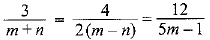Taking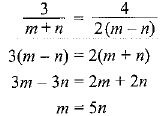...(iii)
Taking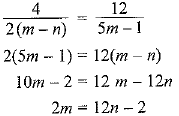...(iv)
Putting m = 5n (iv), we get
2(5n) = 12n - 2
⇒ 2n = 2
⇒ n = l
∴ m = 5(1) = 5 [From (iii)]

Offer running on EduRev: Apply code STAYHOME200 to get INR 200 off on our premium plan EduRev Infinity!

## Mathematics (Maths) Class 10

51 videos|346 docs|103 tests

,

,

,

,

,

,

,

,

,

,

,

,

,

,

,

,

,

,

,

,

,

;